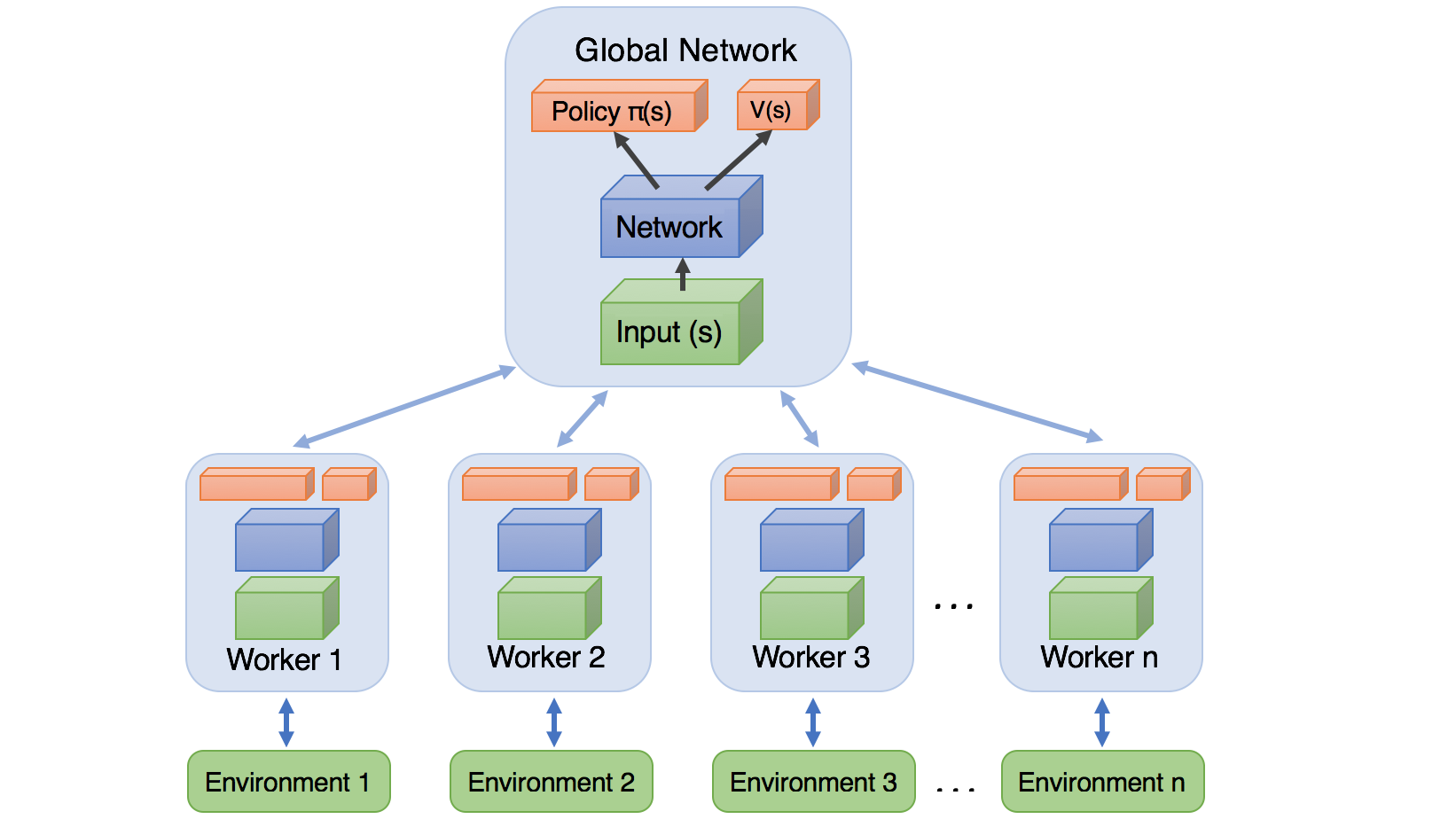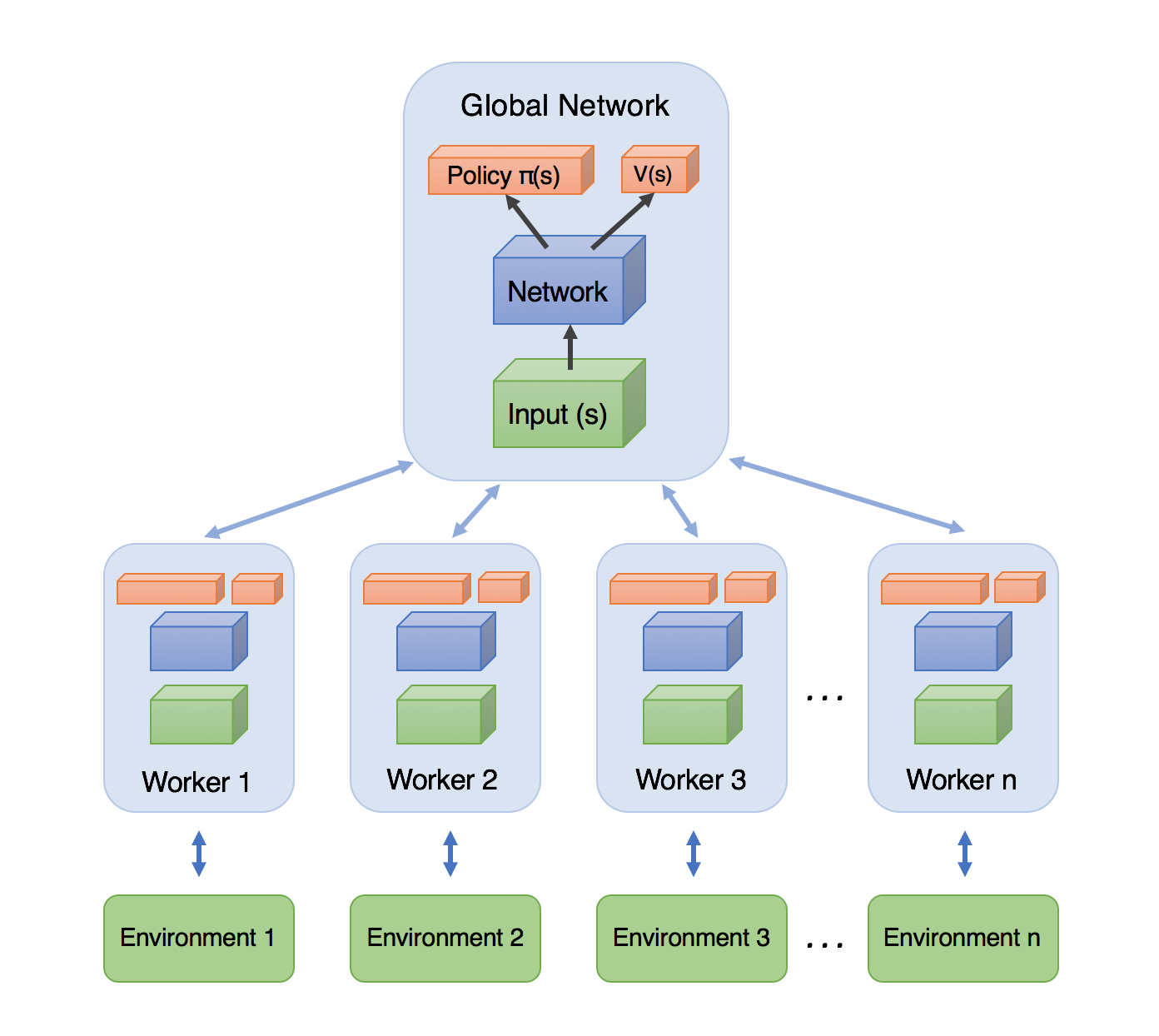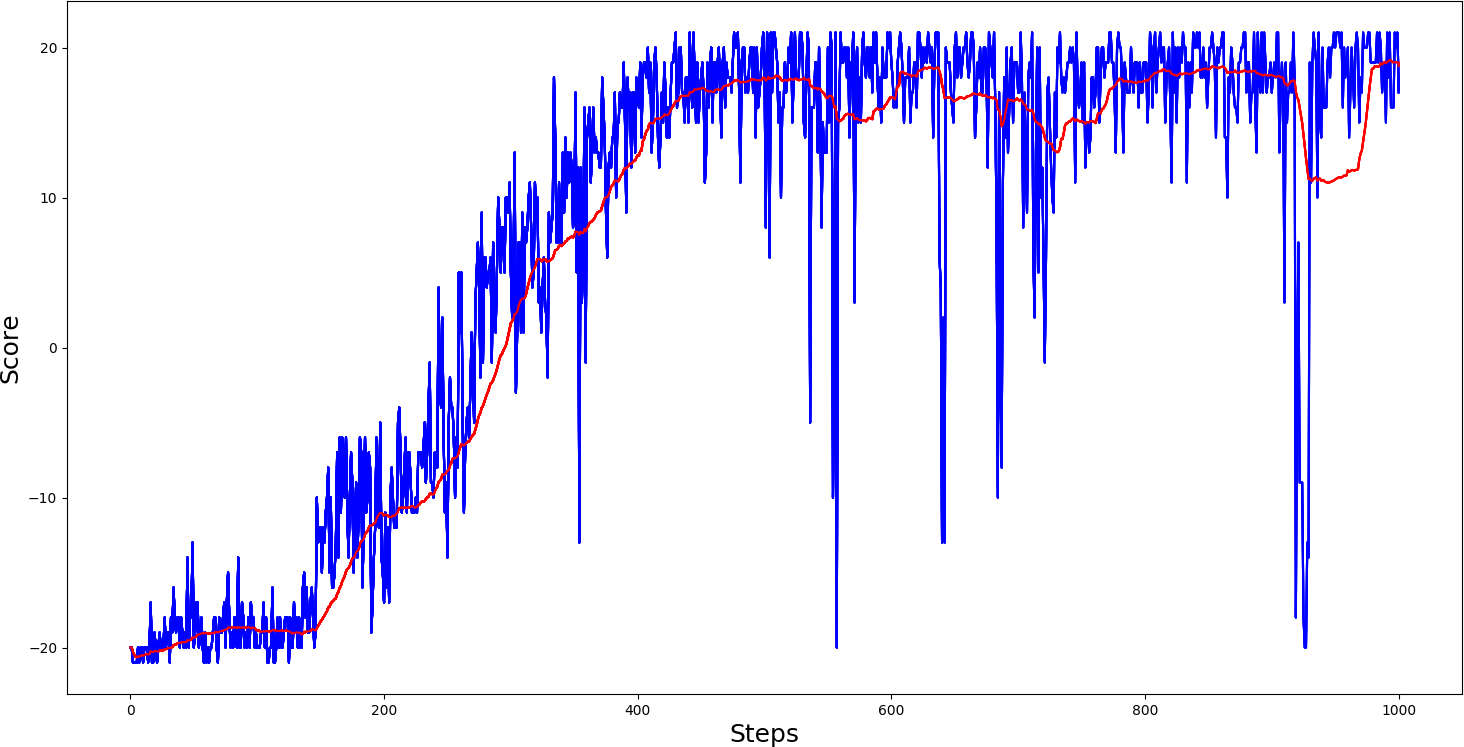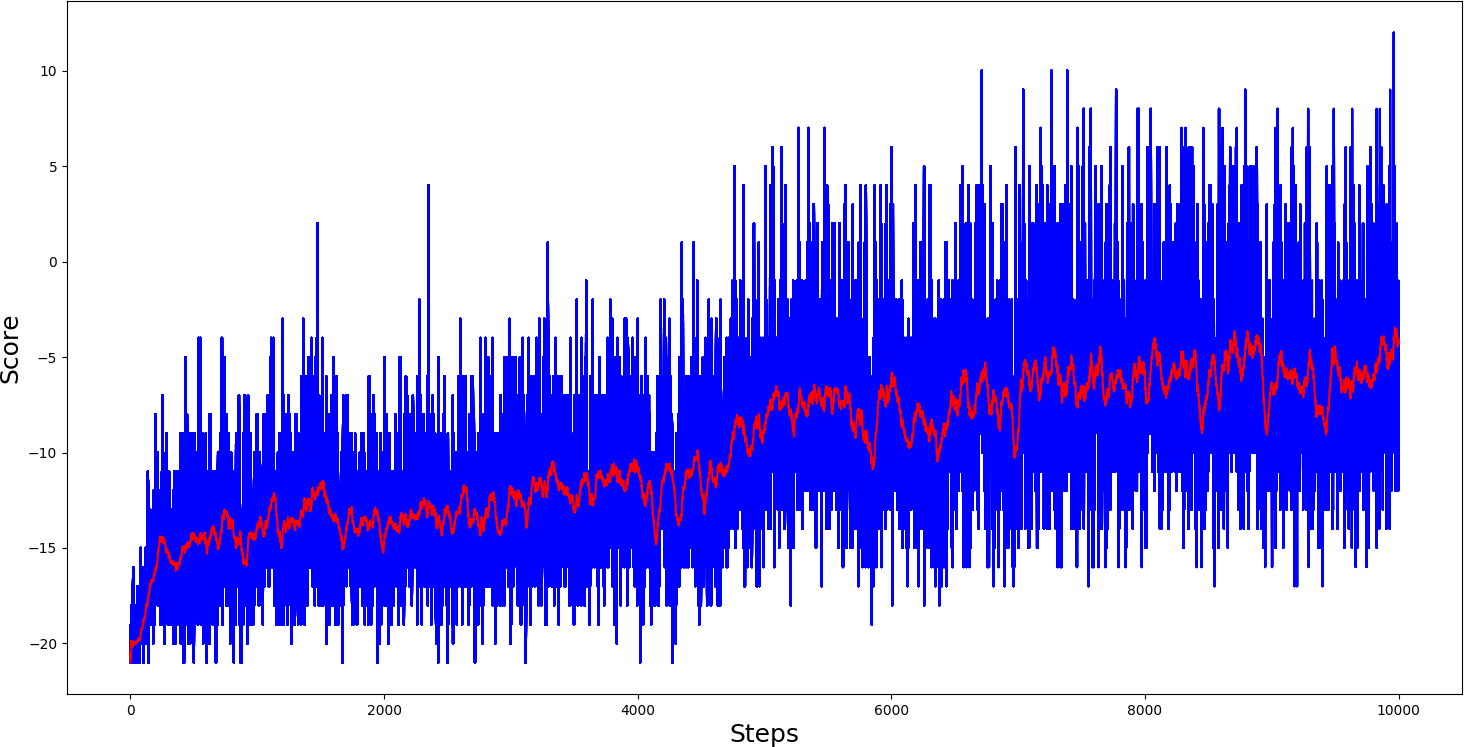# Asynchronous Advantage Actor-Critic (A3C) algorithm

## In this tutorial, I will provide an implementation of the Asynchronous Advantage Actor-Critic (A3C) algorithm in Tensorflow and Keras. We will use it to solve a simple challenge in the Pong environmens

In this tutorial, I will implement the Asynchronous Advantage Actor-Critic (A3C) algorithm in Tensorflow and Keras. We will use it to solve a simple challenge in the Pong environment! If you are new to Deep Learning and Reinforcement Learning, I suggest checking out my previous tutorials before going through this post to understand all the building blocks that will be utilized here. If you have been following the series: thank you! Writing these tutorials, I have learned so much about RL in the past months and am happy to share it with everyone.

So what is A3C? Google's DeepMind group released the A3C algorithm in 2016, and it essentially beat DQN. It was faster, simpler, more robust, and achieved much better scores on the standard Deep RL tasks. On top of all that, it could work in continuous as well as discrete action spaces. A3C became the go-to Deep RL algorithm for new challenging problems with complex state and action spaces.

Asynchronous Advantage Actor-Critic is quite hard to implement. Let's start by breaking down the name and then the mechanics behind the algorithm itself.

• Asynchronous: The algorithm is an asynchronous algorithm where multiple worker agents are trained in parallel, each with their environment. This allows our algorithm to train faster as more workers are training in parallel and attain a more diverse training experience as each worker's experience is independent.
• Advantage: Advantage is a metric to judge how good its actions were and how they turned out. This allows the algorithm to focus on where the network's predictions were lacking. Intuitively, this will enable us to measure the advantage of taking action, following the policy π at the given timestep.
• Actor-Critic: The Actor-Critic aspect of the algorithm uses an architecture that shares layers between the policy and value function.

## How does A3C work?

At a high level, the A3C algorithm uses an asynchronous updating scheme that operates on fixed-length time steps of experience in a continuous environment and batched-length time steps of experience in an episodic environment. It will use these segments to compute estimators of the rewards and the advantage function. Each worker performs the following workflow cycle:

1. Fetch the global network parameters;
2. Interact with the environment by following the local policy for n number of steps;
3. Calculate value and policy loss;
5. Update the global network;
6. Repeat.

With these training steps, we expect to see a linear speedup with the number of agents. However, the number of agents our machine can support is bound by the number of CPU cores available. In addition, A3C can even scale to more than one machine, and some newer research (such as IMPALA) supports scaling it even further. Check out the link for more in-depth information!

## Implementation:

Because I already implemented the A2C agent in my previous tutorial, this part won't affect the agent code that much. We need to make our agents work in parallel. Let's first define what kind of model we'll be using. The master agent will have the global network, and each local worker agent will have a copy of this network in their process.So I use my previous A2C tutorial code as the backbone because we only need to make it work asynchronously. So first, what I do is import the necessary libraries which we will use to make TensorFlow and Keras work in parallel environments:

``````# import needed for threading
import tensorflow as tf
from keras.backend.tensorflow_backend import set_session
import time

# configure Keras and TensorFlow sessions and graph
config = tf.ConfigProto()
config.gpu_options.allow_growth = True
sess = tf.Session(config=config)
set_session(sess)
K.set_session(sess)
graph = tf.get_default_graph()``````

Next, we can move to our initialization step; now, we will use local environment memory instead of global, so we are removing the following lines:

``self.states, self.actions, self.rewards = [], [], []``

Before, we used `self.image_memory`, where we were saving game frames because now we will use this memory locally for every environment, we can remove this memory:

``self.image_memory = np.zeros(self.state_size)``

In the init function, I added `self.episode = 0` parameter, which will be used to track the total count of episodes played through all environments. And defined `self.lock = Lock()` parameters, used to lock all threads to update parameters without other thread interruption.

After creating and compiling our Actor and Critic models, we must create a threaded predict function and save a new graph as global. We do this in the following lines:

``````# make predict function to work while multithreading
self.Actor._make_predict_function()
self.Critic._make_predict_function()

global graph
graph = tf.get_default_graph()``````

If you were following my previous A2C code, we used remember function to create a memory; now, we are removing this function. Next, in remember function, we used to reset our states, action, and rewards memories; we are deleting this line. Instead of using global memory in the replay function, we will give this memory to a function in the following way:

``````def replay(self, states, actions, rewards):
# reshape memory to appropriate shape for training
states = np.vstack(states)
actions = np.vstack(actions)
...``````

What we must change is how we work with every frame. Before we were doing all of this on a global level, now we need to do all these steps for all environments in parallel:

``````def GetImage(self, frame, image_memory):
if image_memory.shape == (1,*self.state_size):
image_memory = np.squeeze(image_memory)

# croping frame to 80x80 size
frame_cropped = frame[35:195:2, ::2,:]
if frame_cropped.shape != self.COLS or frame_cropped.shape != self.ROWS:
# OpenCV resize function
frame_cropped = cv2.resize(frame, (self.COLS, self.ROWS), interpolation=cv2.INTER_CUBIC)

# converting to RGB (numpy way)
frame_rgb = 0.299*frame_cropped[:,:,0] + 0.587*frame_cropped[:,:,1] + 0.114*frame_cropped[:,:,2]

# convert everything to black and white (agent will train faster)
frame_rgb[frame_rgb < 100] = 0
frame_rgb[frame_rgb >= 100] = 255
# converting to RGB (OpenCV way)
#frame_rgb = cv2.cvtColor(frame_cropped, cv2.COLOR_RGB2GRAY)

# dividing by 255 we expresses value to 0-1 representation
new_frame = np.array(frame_rgb).astype(np.float32) / 255.0

# push our data by 1 frame, similar as deq() function work
image_memory = np.roll(image_memory, 1, axis = 0)

# inserting new frame to free space
image_memory[0,:,:] = new_frame

# show image frame
#imshow(image_memory,0)
#imshow(image_memory,1)
#imshow(image_memory,2)
#imshow(image_memory,3)

return np.expand_dims(image_memory, axis=0)

def reset(self, env):
image_memory = np.zeros(self.state_size)
frame = env.reset()
for i in range(self.REM_STEP):
state = self.GetImage(frame, image_memory)
return state

def step(self, action, env, image_memory):
next_state, reward, done, info = env.step(action)
next_state = self.GetImage(next_state, image_memory)
return next_state, reward, done, info``````

So now, when we change our image processing functions, we can work with frames in parallel; they won't be messed up. Now we must change the run function; we will add local memory to this function:

``````def run(self):
for e in range(self.EPISODES):
state = self.reset(self.env)
done, score, SAVING = False, 0, ''
# Instantiate games memory
states, actions, rewards = [], [], []
while not done:
#self.env.render()
# Actor picks an action
action = self.act(state)
# Retrieve new state, reward, and whether the state is terminal
next_state, reward, done, _ = self.step(action, self.env, state)
# Memorize (state, action, reward) for training
states.append(state)
action_onehot = np.zeros([self.action_size])
action_onehot[action] = 1
actions.append(action_onehot)
rewards.append(reward)
# Update current state
state = next_state
score += reward
if done:
average = self.PlotModel(score, e)
# saving best models
if average >= self.max_average:
self.max_average = average
self.save()
SAVING = "SAVING"
else:
SAVING = ""
print("episode: {}/{}, score: {}, average: {:.2f} {}".format(e, self.EPISODES, score, average, SAVING))

self.replay(states, actions, rewards)
# reset training memory
states, actions, rewards = [], [], []

self.env.close()``````

Up to this point, we covered all code we used in the previous tutorial, and we can use it for the A2C model to train. Now it's time to create a simple threading code.

Each with its network and environment, a set of worker agents will be created with the following code. Each of these workers will run on a separate processor thread, so if there will be more workers than threads on CPU, simply worker will play the game slower, keep in mind - this won't give more speed.

``````def train(self, n_threads):
self.env.close()
# Instantiate one environment per thread
envs = [gym.make(self.env_name) for i in range(n_threads)]

daemon=True,
args=(self,
envs[i],

time.sleep(2)
t.start()``````

So, with the above code, we are closing `self.env` we created it before because we just used it to get environment parameters. Now we create a defined number of environments which we target to `train_threading` function; this function will be used to train agents in parallel:

``````def train_threading(self, agent, env, thread):
global graph
with graph.as_default():
while self.episode < self.EPISODES:
# Reset episode
score, done, SAVING = 0, False, ''
state = self.reset(env)
# Instantiate games memory
states, actions, rewards = [], [], []
while not done:
action = agent.act(state)
next_state, reward, done, _ = self.step(action, env, state)

states.append(state)
action_onehot = np.zeros([self.action_size])
action_onehot[action] = 1
actions.append(action_onehot)
rewards.append(reward)

score += reward
state = next_state

self.lock.acquire()
self.replay(states, actions, rewards)
self.lock.release()
# reset training memory
states, actions, rewards = [], [], []

# Update episode count
with self.lock:
average = self.PlotModel(score, self.episode)
# saving best models
if average >= self.max_average:
self.max_average = average
self.save()
SAVING = "SAVING"
else:
SAVING = ""
print("episode: {}/{}, thread: {}, score: {}, average: {:.2f} {}".format(self.episode, self.EPISODES, thread, score, average, SAVING))
if(self.episode < self.EPISODES):
self.episode += 1
env.close() ``````

First, to train our global model in parallel, we are using our defined global graph. When our worker finishes the game episode, and we already have collected game memory, we use `self.lock.acquire()` to lock all treads. We lock threads not to interrupt training by other workers. When finished training, we use `self.lock.release()` and let other threads train the model with their memory. Similar stuff we do to save our plot, we operate with `self.lock`:. All other steps are quite the same.

Once a successful update is made to the global network, the whole process repeats! The worker then resets its network parameters to those of the global network, and the cycle begins again.

To start training agents in parallel with five agents, I use the following code:

``````if __name__ == "__main__":
env_name = 'Pong-v0'
agent = A3CAgent(env_name)
#agent.run() # use as A2C
#agent.test('Pong-v0_A3C_2.5e-05_Actor', 'Pong-v0_A3C_2.5e-05_Critic')``````

So this was quite a long tutorial, and here is the completed code:

``````# Tutorial by www.pylessons.com
# Tutorial written for - Tensorflow 1.15, Keras 2.2.4

import os
import random
import gym
import pylab
import numpy as np
from keras.layers import Input, Dense, Lambda, Add, Conv2D, Flatten
from keras import backend as K
import cv2
import tensorflow as tf
from keras.backend.tensorflow_backend import set_session
import time

# configure Keras and TensorFlow sessions and graph
config = tf.ConfigProto()
config.gpu_options.allow_growth = True
sess = tf.Session(config=config)
set_session(sess)
K.set_session(sess)
graph = tf.get_default_graph()

def OurModel(input_shape, action_space, lr):
X_input = Input(input_shape)

#X = Conv2D(32, 8, strides=(4, 4),padding="valid", activation="elu", data_format="channels_first", input_shape=input_shape)(X_input)
#X = Conv2D(64, 4, strides=(2, 2),padding="valid", activation="elu", data_format="channels_first")(X)
#X = Conv2D(64, 3, strides=(1, 1),padding="valid", activation="elu", data_format="channels_first")(X)
X = Flatten(input_shape=input_shape)(X_input)

X = Dense(512, activation="elu", kernel_initializer='he_uniform')(X)
#X = Dense(256, activation="elu", kernel_initializer='he_uniform')(X)
#X = Dense(64, activation="elu", kernel_initializer='he_uniform')(X)

action = Dense(action_space, activation="softmax", kernel_initializer='he_uniform')(X)
value = Dense(1, kernel_initializer='he_uniform')(X)

Actor = Model(inputs = X_input, outputs = action)
Actor.compile(loss='categorical_crossentropy', optimizer=RMSprop(lr=lr))

Critic = Model(inputs = X_input, outputs = value)
Critic.compile(loss='mse', optimizer=RMSprop(lr=lr))

return Actor, Critic

class A3CAgent:
# Actor-Critic Main Optimization Algorithm
def __init__(self, env_name):
# Initialization
# Environment and PPO parameters
self.env_name = env_name
self.env = gym.make(env_name)
self.action_size = self.env.action_space.n
self.EPISODES, self.episode, self.max_average = 10000, 0, -21.0 # specific for pong
self.lock = Lock()
self.lr = 0.000025

self.ROWS = 80
self.COLS = 80
self.REM_STEP = 4

# Instantiate plot memory
self.scores, self.episodes, self.average = [], [], []

self.Save_Path = 'Models'
self.state_size = (self.REM_STEP, self.ROWS, self.COLS)

if not os.path.exists(self.Save_Path): os.makedirs(self.Save_Path)
self.path = '{}_A3C_{}'.format(self.env_name, self.lr)
self.Model_name = os.path.join(self.Save_Path, self.path)

# Create Actor-Critic network model
self.Actor, self.Critic = OurModel(input_shape=self.state_size, action_space = self.action_size, lr=self.lr)

# make predict function to work while multithreading
self.Actor._make_predict_function()
self.Critic._make_predict_function()

global graph
graph = tf.get_default_graph()

def act(self, state):
# Use the network to predict the next action to take, using the model
prediction = self.Actor.predict(state)
action = np.random.choice(self.action_size, p=prediction)
return action

def discount_rewards(self, reward):
# Compute the gamma-discounted rewards over an episode
gamma = 0.99    # discount rate
discounted_r = np.zeros_like(reward)
for i in reversed(range(0,len(reward))):
if reward[i] != 0: # reset the sum, since this was a game boundary (pong specific!)

discounted_r -= np.mean(discounted_r) # normalizing the result
discounted_r /= np.std(discounted_r) # divide by standard deviation
return discounted_r

def replay(self, states, actions, rewards):
# reshape memory to appropriate shape for training
states = np.vstack(states)
actions = np.vstack(actions)

# Compute discounted rewards
discounted_r = self.discount_rewards(rewards)

# Get Critic network predictions
value = self.Critic.predict(states)[:, 0]
# training Actor and Critic networks
self.Critic.fit(states, discounted_r, epochs=1, verbose=0)

def save(self):
self.Actor.save(self.Model_name + '_Actor.h5')
#self.Critic.save(self.Model_name + '_Critic.h5')

pylab.figure(figsize=(18, 9))
def PlotModel(self, score, episode):
self.scores.append(score)
self.episodes.append(episode)
self.average.append(sum(self.scores[-50:]) / len(self.scores[-50:]))
if str(episode)[-2:] == "00":# much faster than episode % 100
pylab.plot(self.episodes, self.scores, 'b')
pylab.plot(self.episodes, self.average, 'r')
pylab.ylabel('Score', fontsize=18)
pylab.xlabel('Steps', fontsize=18)
try:
pylab.savefig(self.path+".png")
except OSError:
pass

return self.average[-1]

def imshow(self, image, rem_step=0):
cv2.imshow(self.Model_name+str(rem_step), image[rem_step,...])
if cv2.waitKey(25) & 0xFF == ord("q"):
cv2.destroyAllWindows()
return

def GetImage(self, frame, image_memory):
if image_memory.shape == (1,*self.state_size):
image_memory = np.squeeze(image_memory)

# croping frame to 80x80 size
frame_cropped = frame[35:195:2, ::2,:]
if frame_cropped.shape != self.COLS or frame_cropped.shape != self.ROWS:
# OpenCV resize function
frame_cropped = cv2.resize(frame, (self.COLS, self.ROWS), interpolation=cv2.INTER_CUBIC)

# converting to RGB (numpy way)
frame_rgb = 0.299*frame_cropped[:,:,0] + 0.587*frame_cropped[:,:,1] + 0.114*frame_cropped[:,:,2]

# convert everything to black and white (agent will train faster)
frame_rgb[frame_rgb < 100] = 0
frame_rgb[frame_rgb >= 100] = 255
# converting to RGB (OpenCV way)
#frame_rgb = cv2.cvtColor(frame_cropped, cv2.COLOR_RGB2GRAY)

# dividing by 255 we expresses value to 0-1 representation
new_frame = np.array(frame_rgb).astype(np.float32) / 255.0

# push our data by 1 frame, similar as deq() function work
image_memory = np.roll(image_memory, 1, axis = 0)

# inserting new frame to free space
image_memory[0,:,:] = new_frame

# show image frame
#self.imshow(image_memory,0)
#self.imshow(image_memory,1)
#self.imshow(image_memory,2)
#self.imshow(image_memory,3)

return np.expand_dims(image_memory, axis=0)

def reset(self, env):
image_memory = np.zeros(self.state_size)
frame = env.reset()
for i in range(self.REM_STEP):
state = self.GetImage(frame, image_memory)
return state

def step(self, action, env, image_memory):
next_state, reward, done, info = env.step(action)
next_state = self.GetImage(next_state, image_memory)
return next_state, reward, done, info

def run(self):
for e in range(self.EPISODES):
state = self.reset(self.env)
done, score, SAVING = False, 0, ''
# Instantiate or reset games memory
states, actions, rewards = [], [], []
while not done:
#self.env.render()
# Actor picks an action
action = self.act(state)
# Retrieve new state, reward, and whether the state is terminal
next_state, reward, done, _ = self.step(action, self.env, state)
# Memorize (state, action, reward) for training
states.append(state)
action_onehot = np.zeros([self.action_size])
action_onehot[action] = 1
actions.append(action_onehot)
rewards.append(reward)
# Update current state
state = next_state
score += reward
if done:
average = self.PlotModel(score, e)
# saving best models
if average >= self.max_average:
self.max_average = average
self.save()
SAVING = "SAVING"
else:
SAVING = ""
print("episode: {}/{}, score: {}, average: {:.2f} {}".format(e, self.EPISODES, score, average, SAVING))

self.replay(states, actions, rewards)
# close environemnt when finish training
self.env.close()

self.env.close()
# Instantiate one environment per thread
envs = [gym.make(self.env_name) for i in range(n_threads)]

daemon=True,
args=(self,
envs[i],

time.sleep(2)
t.start()

global graph
with graph.as_default():
while self.episode < self.EPISODES:
# Reset episode
score, done, SAVING = 0, False, ''
state = self.reset(env)
# Instantiate or reset games memory
states, actions, rewards = [], [], []
while not done:
action = agent.act(state)
next_state, reward, done, _ = self.step(action, env, state)

states.append(state)
action_onehot = np.zeros([self.action_size])
action_onehot[action] = 1
actions.append(action_onehot)
rewards.append(reward)

score += reward
state = next_state

self.lock.acquire()
self.replay(states, actions, rewards)
self.lock.release()

# Update episode count
with self.lock:
average = self.PlotModel(score, self.episode)
# saving best models
if average >= self.max_average:
self.max_average = average
self.save()
SAVING = "SAVING"
else:
SAVING = ""
print("episode: {}/{}, thread: {}, score: {}, average: {:.2f} {}".format(self.episode, self.EPISODES, thread, score, average, SAVING))
if(self.episode < self.EPISODES):
self.episode += 1
env.close()

def test(self, Actor_name, Critic_name):
for e in range(100):
state = self.reset(self.env)
done = False
score = 0
while not done:
action = np.argmax(self.Actor.predict(state))
state, reward, done, _ = self.step(action, self.env, state)
score += reward
if done:
print("episode: {}/{}, score: {}".format(e, self.EPISODES, score))
break
self.env.close()

if __name__ == "__main__":
env_name = 'Pong-v0'
agent = A3CAgent(env_name)
#agent.run() # use as A2C
#agent.test('Pong-v0_A3C_2.5e-05_Actor.h5', 'Pong-v0_A3C_2.5e-05_Critic.h5')``````

Same as before, you probably want to see my training results with the Pong game, right? Actually, it was the same, but instead of training the agent for a few days, I trained it in half a day with five workers. With the asynchronous method, we can train agents few times faster. It's easier for us to test different model hyper-parameters or see if our agent is improving. So, these are the results of my A3C agent playing PongDeterministic-v4:As you can see, our agent in the PongDeterministic-v4 environment achieved the best average score in around 500th step, comparing A3C with A2C results are pretty similar, the difference is time, to train agent for 1000 steps took only a few hours. A similar win is with the Pong-v0 environment because I won an advantage in time. I thought that I would try to train the agent for 20000 steps and will see if it improves; here is the training curve:So we can see that after 10k steps, our agent was still improving, so if we trained it further, it would improve slightly more, but slower and slower. So we need to change something to achieve results faster.

## Conclusion:

I hope this tutorial has been helpful to those who are new to Asynchronous Reinforcement learning! Now you can build almost any reinforcement agent which could be trained in parallel. So there is one more tutorial coming, one of the most popular Proximal Policy Optimization (PPO) algorithm, but I will do it the same way - in parallel. I hope you understand how A3C works because I will use it as a backbone. See you in the next tutorial. Code uploaded on: GitHub.